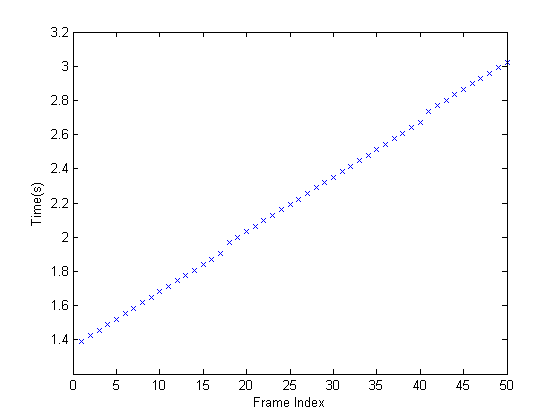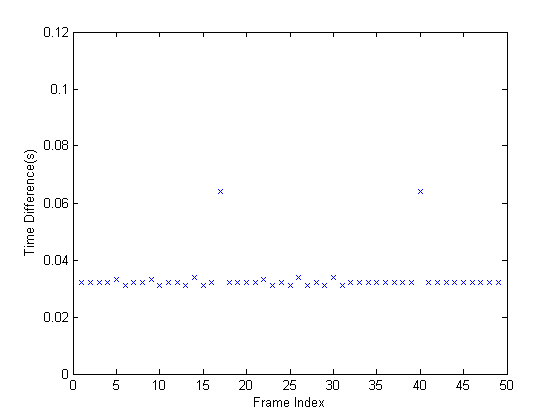# Determining the Rate of Acquisition

This example shows how to use the timestamps provided by the GETDATA function, and estimate the device frame rate using MATLAB® functions.

### Step 1: Access and Configure a Device.

Create a video input object and access its video source object to configure the desired acquisition rate. The acquisition rate is determined by the value of the device specific FrameRate property of the video source object.

Note, since FrameRate is a device specific property, not all devices may support it.

```% Access an image acquisition device. vidobj = videoinput('winvideo', 1); % Configure the number of frames to log. vidobj.FramesPerTrigger = 50; % Skip the first few frames the device provides % before logging data. vidobj.TriggerFrameDelay = 5; % Access the device's video source. src = getselectedsource(vidobj); % Determine the device specific frame rates (frames per second) available. frameRates = set(src, 'FrameRate') ```
```frameRates = '30.0000' '24.0000' '8.0000' ```
```% Configure the device's frame rate to the highest available setting. src.FrameRate = frameRates{1}; actualRate = str2num( frameRates{1} ) ```
```actualRate = 30 ```

### Step 2: Log and Retrieve Data.

Initiate the acquisition and retrieve the logged frames and timestamps.

```% Start the acquisition. start(vidobj) % Wait for data logging to end before retrieving data. Set the wait time % to be equal to the expected time to acquire the number of frames % specified plus a little buffer time to accommodate overhead. waittime = actualRate * (vidobj.FramesPerTrigger + vidobj.TriggerFrameDelay) + 5; wait(vidobj, waittime); % Retrieve the data and timestamps. [frames, timeStamp] = getdata(vidobj); ```

### Step 3: Calculate the Acquisition Rate.

By plotting each frame's timestamp, one can verify that the rate of acquisition is constant.

```% Graph frames vs time. plot(timeStamp,'x') xlabel('Frame Index') ylabel('Time(s)') ```The average time difference can also be determined to compare to the expected acquisition rate.

```% Find the time difference between frames. diffFrameTime = diff(timeStamp); % Graph the time differences. plot(diffFrameTime, 'x'); xlabel('Frame Index') ylabel('Time Difference(s)') ylim([0 .12]) ``````% Find the average time difference between frames. avgTime = mean(diffFrameTime) ```
```avgTime = 0.0333 ```
```% Determine the experimental frame rate. expRate = 1/avgTime ```
```expRate = 30.0245 ```

Comparing the time difference between the experimental and the known frame rate, the percent error can be calculated. Since a generic USB web camera is being used as the acquisition device, it is to be expected that the actual device frame rate will fluctuate.

```% Determine the percent error between the determined and actual frame rate. diffRates = abs(actualRate - expRate) ```
```diffRates = 0.0245 ```
```percentError = (diffRates/actualRate) * 100 ```
```percentError = 0.0817 ```
```% Once the video input object is no longer needed, delete % it and clear it from the workspace. delete(vidobj) clear vidobj ```
﻿
##### Support